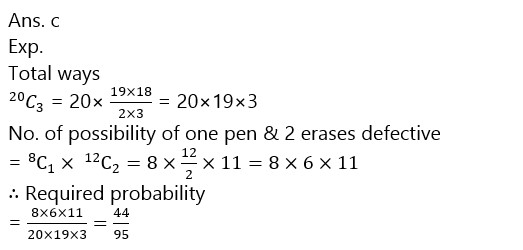# RBI Assistant Pre 2023 Quantitative Aptitude Quiz -15

## RBI Assistant Pre 2023 Quantitative Aptitude

Quantitative Aptitude is the most significant part of almost all competitive exams. Many candidates find difficulty in solving Quantitative Aptitude Questions. The only way to ace this section is to practice as many questions as possible. And, to help you practice more and more questions, we have come up with the RBI Assistant Pre 2023 Quantitative Aptitude Quiz. This RBI Assistant Pre 2023 Quantitative Aptitude Quiz is completely Free. This RBI Assistant Pre 2023 Quantitative Aptitude Quiz includes a variety of questions that are asked in exams. Candidates will be provided with a detailed solution for each question in this RBI Assistant Pre 2023 Quantitative Aptitude Quiz. This RBI Assistant Pre 2023 Quantitative Aptitude Quiz will assist candidates in better preparing for upcoming exams.

1. I. 9x² – 24x + 7 = 0

3y² + 23y – 8 = 0

(a) if x>y

(b) if x≥y

(c) if x<y

(d) if x ≤y

(e) if x = y or no relation can be established between x and y1. I. 4x² + 13x + 3 = 0

y² + 16y + 63 = 0

(a) if x>y

(b) if x≥y

(c) if x<y

(d) if x ≤y

(e) if x = y or no relation can be established between x and y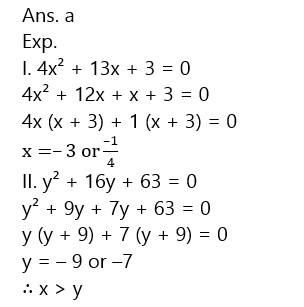1. I. 15x² – 16x + 4 = 0

2y² – 15y + 7 = 0

(a) if x>y

(b) if x≥y

(c) if x<y

(d) if x ≤y

(e) if x = y or no relation can be established between x and y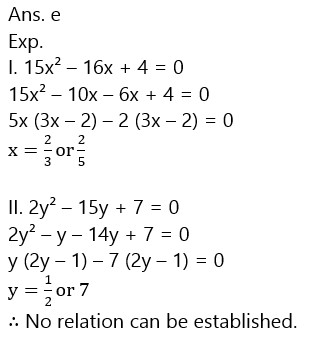1. I. 7x + 2y = 31

4x + 3y = 8

(a) if x>y

(b) if x≥y

(c) if x<y

(d) if x ≤y

(e) if x = y or no relation can be established between x and y1. I. 4x² – 37x – 30 = 0

y² – 13y + 12 = 0

(a) if x>y

(b) if x≥y

(c) if x<y

(d) if x ≤y

(e) if x = y or no relation can be established between x and y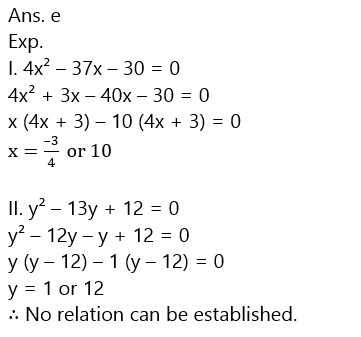1. If speed of boat upstream is twice the speed of stream and time taken by boat to travel between two points and return back is 8 hours. Then speed of boat in still water is what percent of speed of stream if distance between the two points is given 64 km?

(a) 300%

(b) 200%

(c) 325%

(d) 250%

(e) 350%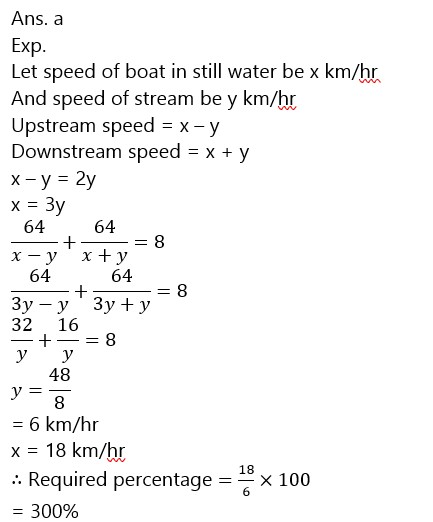1. In how many different ways can the letters of the word ALLAHABAD be arranged so that the vowel occupy even places?

(a) 80

(b) 60

(c) 120

(d) 75

(e) 50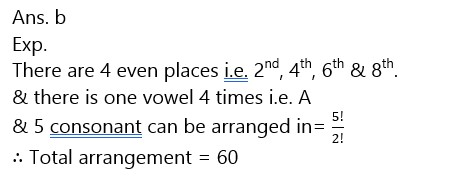1. In a class there are 27 students in which some are boys and some are girls. If a student is selected at random from the class for monitor then probability of selected student being a girl is 1/3. Then how many boys are there in class?

(a) 12

(b) 9

(c) 7

(d) 24

(e) 18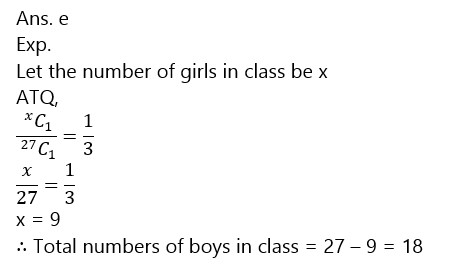1. The height of cone is equal to the length of rectangle whose area is equal to 120cm² and length of rectangle is 20% more than breadth and radius of cone is 1/3rd of the height of cone. Then find volume of cone? (in cm³)

(a) 58 π

(b) 52 π

(c) 48 π

(d) 64 π

(e) 72 π1. There are 12 erasers & 8 pens in a box. If three of them are defective then what is the probability that one of the three is pen & other two are erasers?

(a)   44/93

(b)  41/93

(c)   44/95

(d)  41/95

(e)  42/97